# 看一遍就理解：动态规划详解

29,134

### 前言

• 什么是动态规划？
• 动态规划的核心思想
• 一个例子走进动态规划
• 动态规划的解题套路
• leetcode案例分析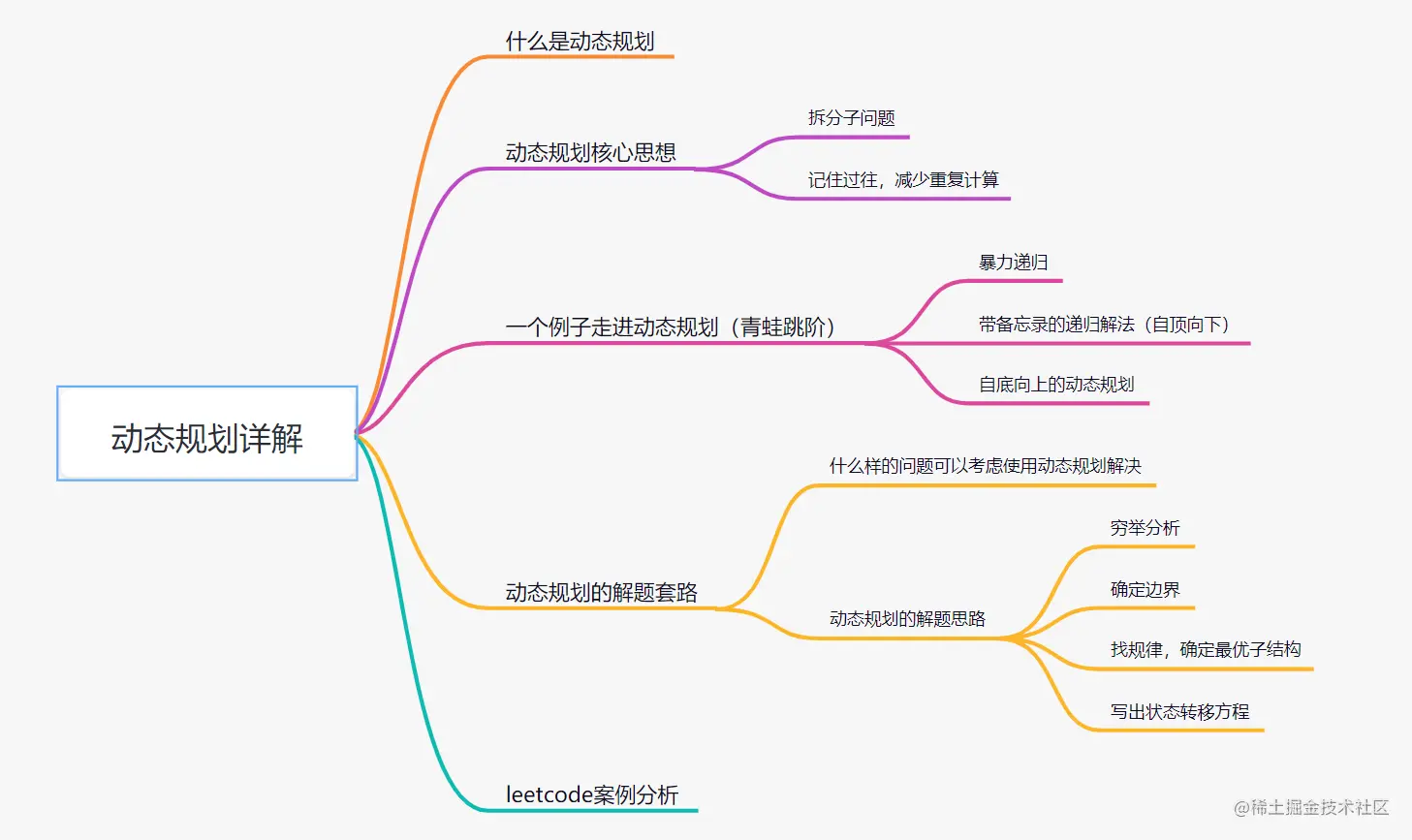### 什么是动态规划？

dynamic programming is a method for solving a complex problem by breaking it down into a collection of simpler subproblems.

### 动态规划核心思想• A ： "1+1+1+1+1+1+1+1 =？"
• A ： "上面等式的值是多少"
• B ： 计算 "8"
• A : 在上面等式的左边写上 "1+" 呢？
• A : "此时等式的值为多少"
• B : 很快得出答案 "9"
• A : "你怎么这么快就知道答案了"
• A : "只要在8的基础上加1就行了"
• A : "所以你不用重新计算，因为你记住了第一个等式的值为8!动态规划算法也可以说是 '记住求过的解来节省时间'"

### 一个例子带你走进动态规划 -- 青蛙跳阶问题

#### 暴力递归

leetcode原题：一只青蛙一次可以跳上1级台阶，也可以跳上2级台阶。求该青蛙跳上一个 10 级的台阶总共有多少种跳法。

• 要想跳到第10级台阶，要么是先跳到第9级，然后再跳1级台阶上去;要么是先跳到第8级，然后一次迈2级台阶上去。
• 同理，要想跳到第9级台阶，要么是先跳到第8级，然后再跳1级台阶上去;要么是先跳到第7级，然后一次迈2级台阶上去。
• 要想跳到第8级台阶，要么是先跳到第7级，然后再跳1级台阶上去;要么是先跳到第6级，然后一次迈2级台阶上去。

``````f（10） = f（9）+f(8)
f (9)  = f(8) + f(7)
f (8)  = f(7) + f(6)
...
f(3) = f(2) + f(1)

``````

• 当只有2级台阶时，有两种跳法，第一种是直接跳两级，第二种是先跳一级，然后再跳一级。即f(2) = 2;
• 当只有1级台阶时，只有一种跳法，即f（1）= 1；

``````class Solution {
public int numWays(int n) {
if(n == 1){
return 1;
}
if(n == 2){
return 2;
}
return numWays(n-1) + numWays(n-2);
}
}
``````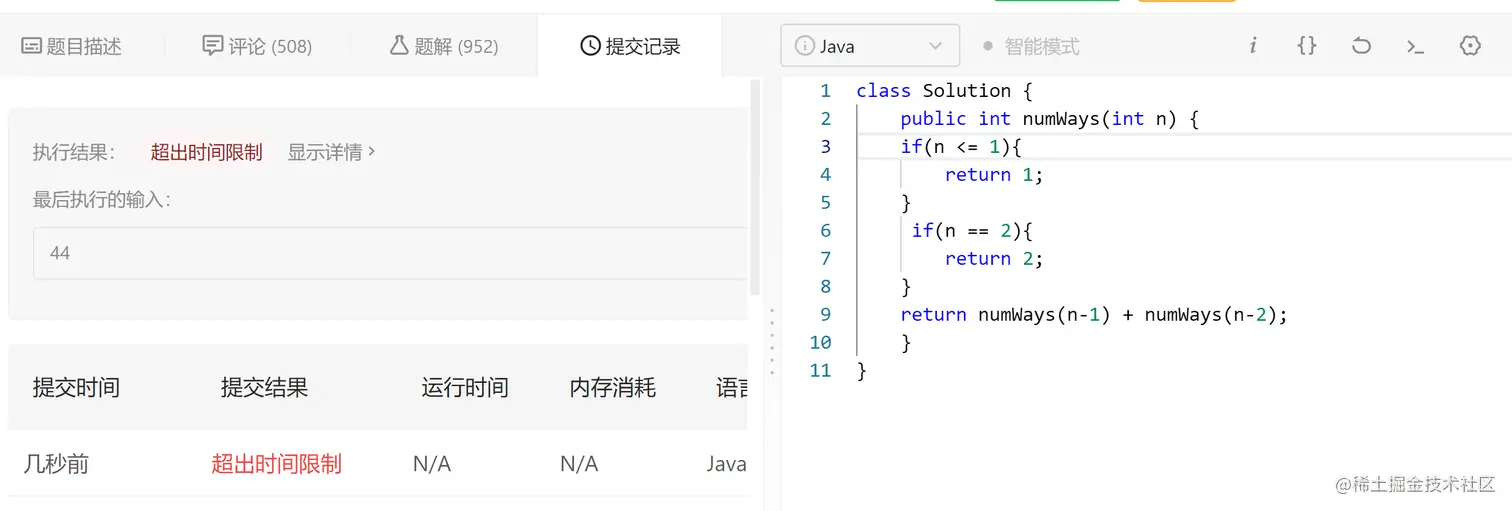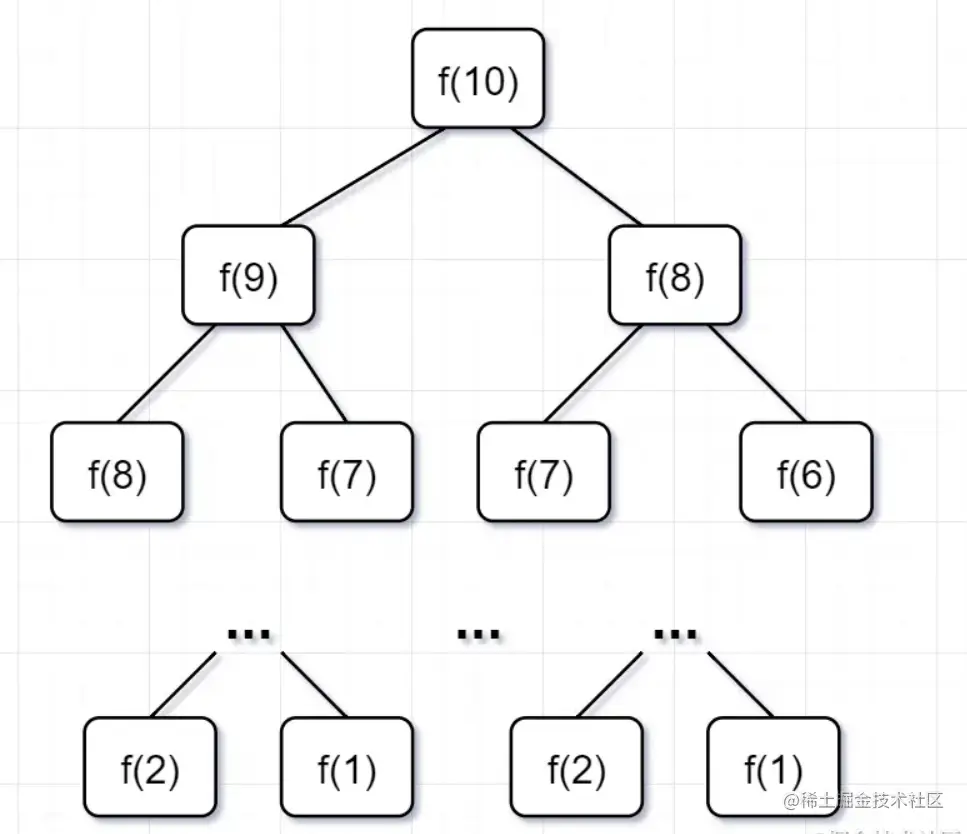• 要计算原问题 f(10)，就需要先计算出子问题 f(9) 和 f(8)
• 然后要计算 f(9)，又要先算出子问题 f(8) 和 f(7)，以此类推。
• 一直到 f(2) 和 f(1），递归树才终止。

``````递归时间复杂度 = 解决一个子问题时间*子问题个数
``````
• 一个子问题时间 = f（n-1）+f（n-2），也就是一个加法的操作，所以复杂度是 O(1)；
• 问题个数 = 递归树节点的总数，递归树的总节点 = 2^n-1，所以是复杂度O(2^n)。

#### 带备忘录的递归解法（自顶向下）

• 第一步，f（10）= f(9) + f(8)，f(9) 和f（8）都需要计算出来，然后再加到备忘录中，如下：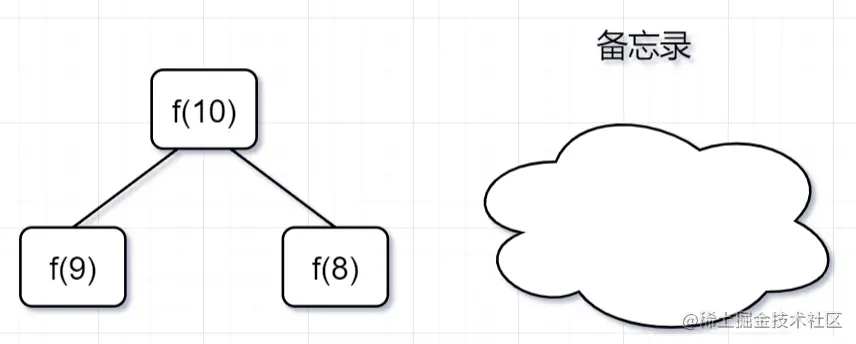• 第二步， f(9) = f（8）+ f（7），f（8）= f（7）+ f(6), 因为 f(8) 已经在备忘录中啦，所以可以省掉，f(7),f（6）都需要计算出来，加到备忘录中~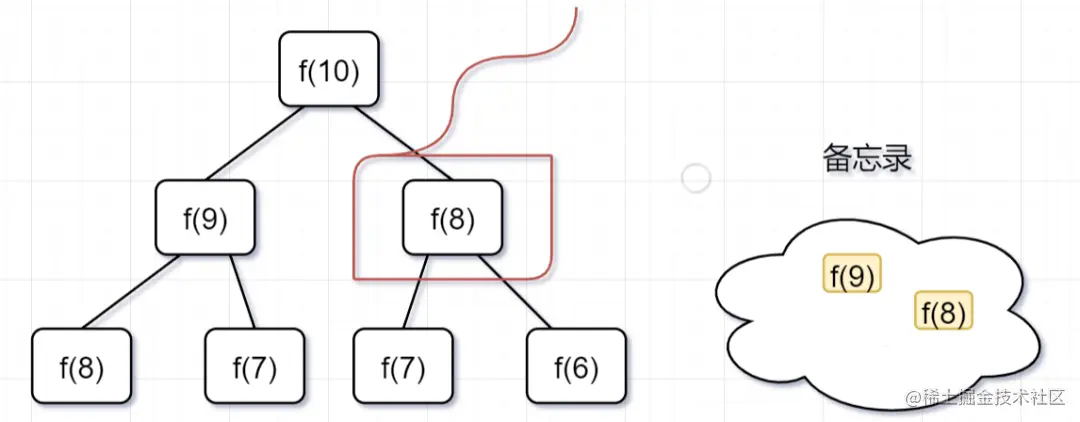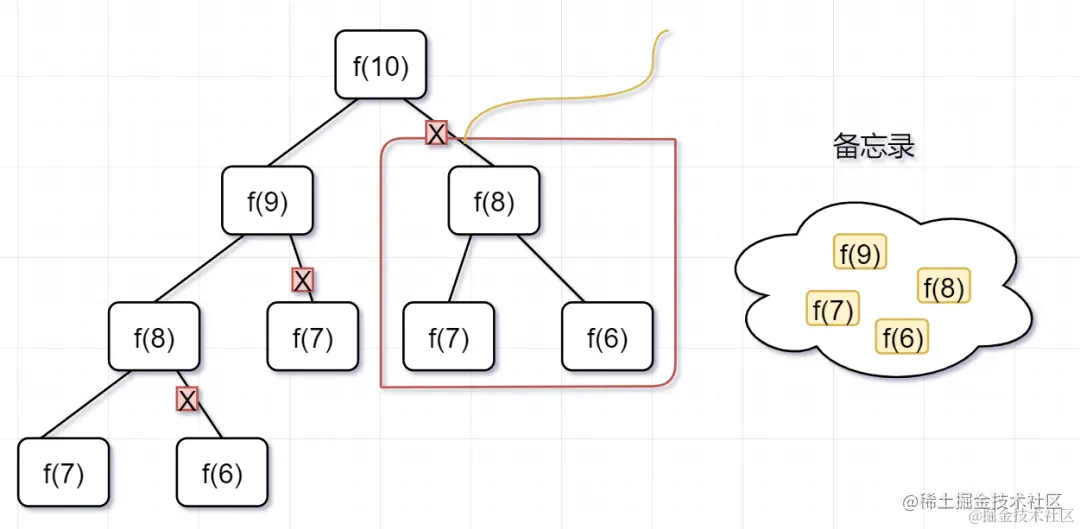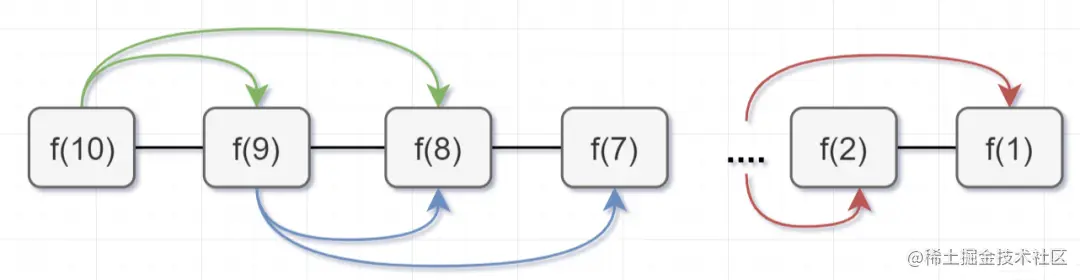``````public class Solution {
//使用哈希map，充当备忘录的作用
Map<Integer, Integer> tempMap = new HashMap();
public int numWays(int n) {
// n = 0 也算1种
if (n == 0) {
return 1;
}
if (n <= 2) {
return n;
}
//先判断有没计算过，即看看备忘录有没有
if (tempMap.containsKey(n)) {
//备忘录有，即计算过，直接返回
return tempMap.get(n);
} else {
// 备忘录没有，即没有计算过，执行递归计算,并且把结果保存到备忘录map中，对1000000007取余（这个是leetcode题目规定的）
tempMap.put(n, (numWays(n - 1) + numWays(n - 2)) % 1000000007);
return tempMap.get(n);
}
}
}
``````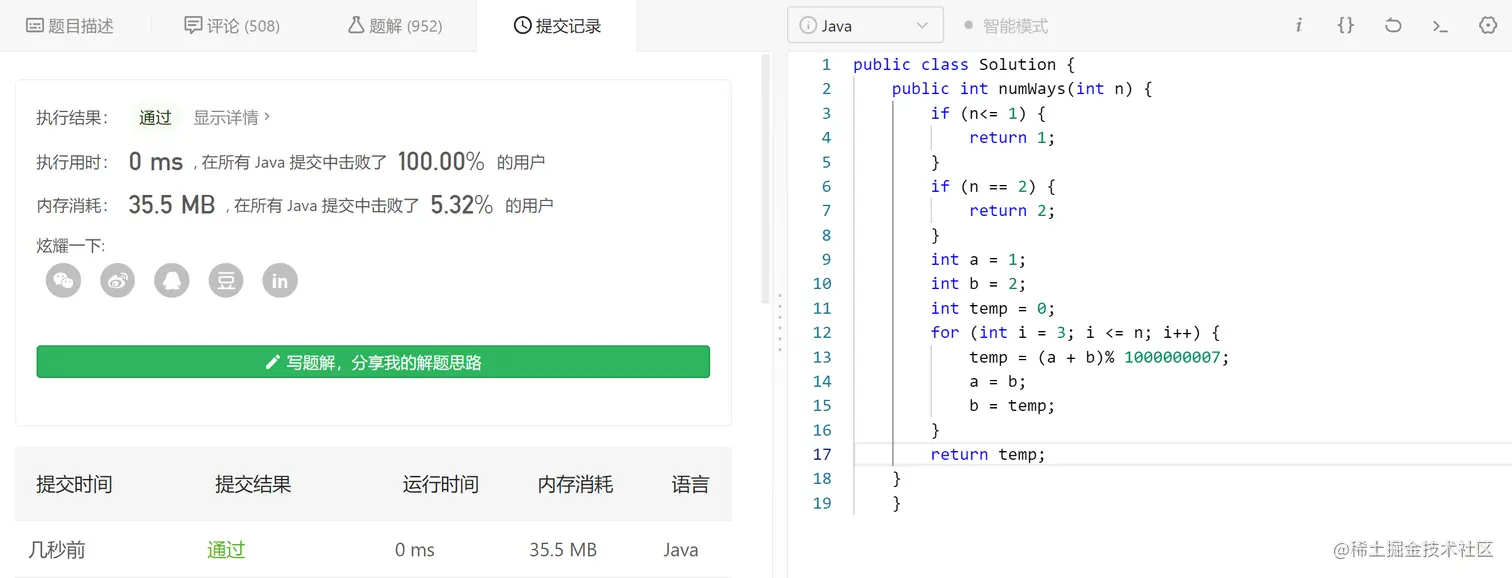#### 自底向上的动态规划

• 带备忘录的递归，是从f(10)往f(1）方向延伸求解的，所以也称为自顶向下的解法。
• 动态规划从较小问题的解，由交叠性质，逐步决策出较大问题的解，它是从f(1)往f(10）方向，往上推求解，所以称为自底向上的解法。

• f(n-1)和f(n-2) 称为 f(n) 的最优子结构
• f(n)= f（n-1）+f（n-2）就称为状态转移方程
• f(1) = 1, f(2) = 2 就是边界啦
• 比如f(10)= f(9)+f(8),f(9) = f(8) + f(7) ,f(8)就是重叠子问题。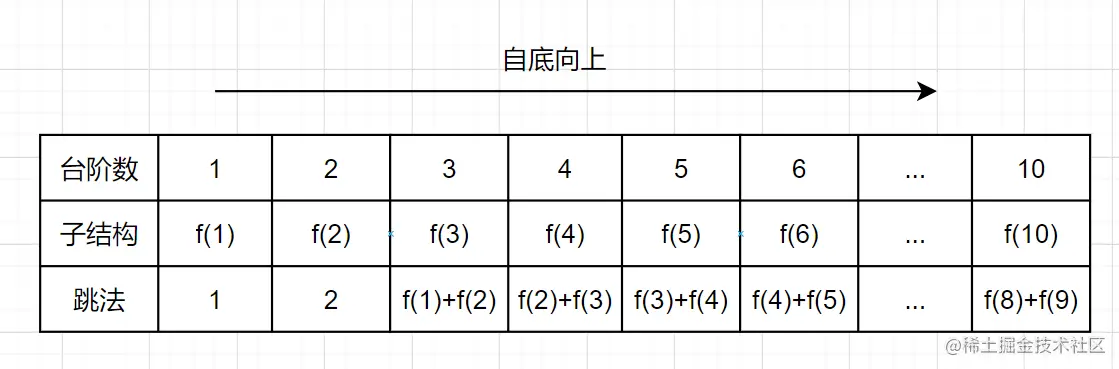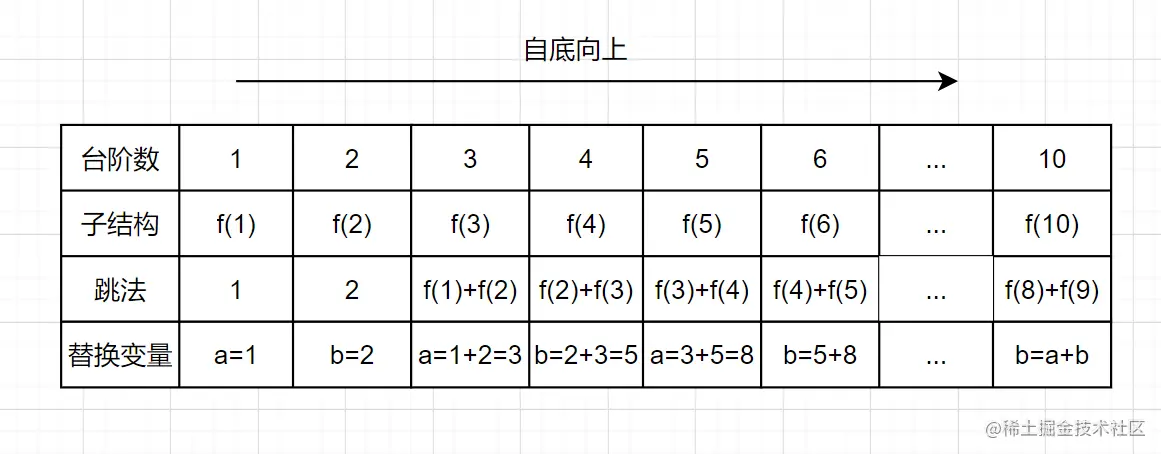``````public class Solution {
public int numWays(int n) {
if (n<= 1) {
return 1;
}
if (n == 2) {
return 2;
}
int a = 1;
int b = 2;
int temp = 0;
for (int i = 3; i <= n; i++) {
temp = (a + b)% 1000000007;
a = b;
b = temp;
}
return temp;
}
}
``````

### 动态规划的解题思路

• 穷举分析
• 确定边界
• 找出规律，确定最优子结构
• 写出状态转移方程

#### 1. 穷举分析

• 当台阶数是1的时候，有一种跳法，f（1） =1
• 当只有2级台阶时，有两种跳法，第一种是直接跳两级，第二种是先跳一级，然后再跳一级。即f(2) = 2;
• 当台阶是3级时，想跳到第3级台阶，要么是先跳到第2级，然后再跳1级台阶上去，要么是先跳到第 1级，然后一次迈 2 级台阶上去。所以f(3) = f(2) + f(1) =3
• 当台阶是4级时，想跳到第3级台阶，要么是先跳到第3级，然后再跳1级台阶上去，要么是先跳到第 2级，然后一次迈 2 级台阶上去。所以f(4) = f(3) + f(2) =5
• 当台阶是5级时......#### 3. 找规律，确定最优子结构

n>=3时，已经呈现出规律 f(n) = f(n-1) + f(n-2) ，因此，f(n-1)和f(n-2) 称为 f(n) 的最优子结构。什么是最优子结构？有这么一个解释：

#### 4， 写出状态转移方程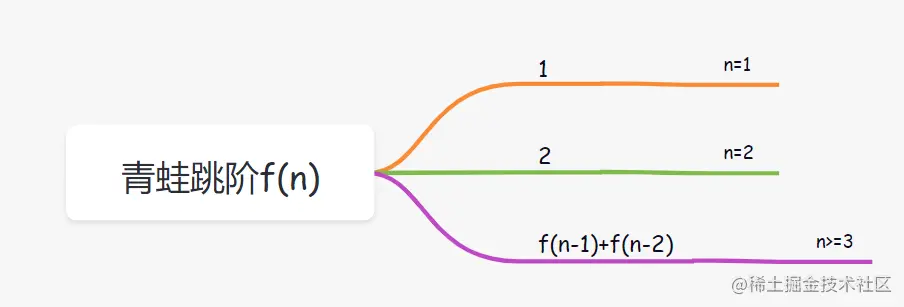#### 5. 代码实现

``````dp[...] = 边界值
for(状态1 ：所有状态1的值){
for(状态2 ：所有状态2的值){
for(...){
//状态转移方程
dp[状态1][状态2][...] = 求最值
}
}
}
``````

### leetcode案例分析

``````输入：nums = [10,9,2,5,3,7,101,18]

``````

``````输入：nums = [0,1,0,3,2,3]

``````

• 穷举分析
• 确定边界
• 找规律，确定最优子结构
• 状态转移方程

#### 1.穷举分析

##### 自顶向上的穷举

• 当nums只有一个元素10时，最长递增子序列是,长度是1.
• 当nums需要加入一个元素9时，最长递增子序列是或者,长度是1。
• 当nums再加入一个元素2时，最长递增子序列是或者或者,长度是1。
• 当nums再加入一个元素5时，最长递增子序列是[2,5],长度是2。
• 当nums再加入一个元素3时，最长递增子序列是[2,5]或者[2,3],长度是2。
• 当nums再加入一个元素7时，,最长递增子序列是[2,5,7]或者[2,3,7],长度是3。
• 当nums再加入一个元素101时，最长递增子序列是[2,5,7,101]或者[2,3,7,101],长度是4。
• 当nums再加入一个元素18时，最长递增子序列是[2,5,7,101]或者[2,3,7,101]或者[2,5,7,18]或者[2,3,7,18],长度是4。
• 当nums再加入一个元素7时,最长递增子序列是[2,5,7,101]或者[2,3,7,101]或者[2,5,7,18]或者[2,3,7,18],长度是4.
##### 分析找规律，拆分子问题

``````原问题数组nums[i]的最长递增子序列 = 子问题数组nums[i-1]的最长递增子序列/nums[i]结尾的最长递增子序列
``````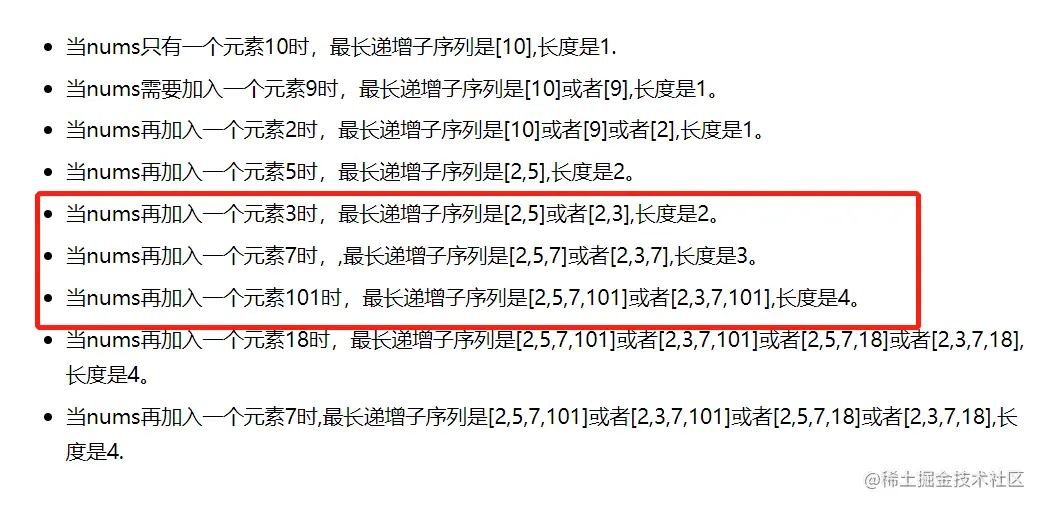nums[i]的最长递增子序列，不就是从以数组num[i]每个元素结尾的最长子序列集合，取元素最多（也就是长度最长）那个嘛，所以原问题，我们转化成求出以数组nums每个元素结尾的最长子序列集合，再取最大值嘛。哈哈，想到这，我们就可以用dp[i]表示以num[i]这个数结尾的最长递增子序列的长度啦，然后再来看看其中的规律：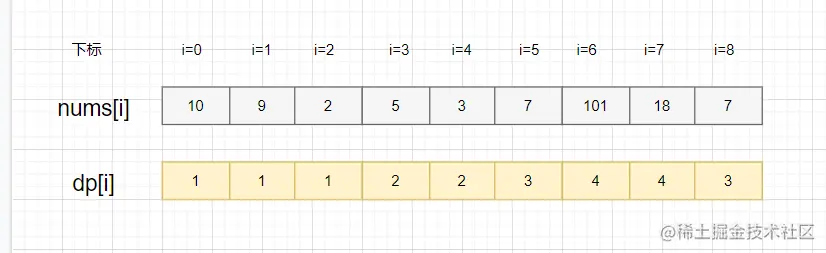• nums=5,以`5`结尾的最长子序列就是`[2,5]`,因为从数组下标`0到3`遍历，只找到了子序列```5`小，所以就是`+`啦，即`dp=2`
• nums=3,以`3`结尾的最长子序列就是`[2,3]`,因为从数组下标`0到4`遍历，只找到了子序列```3`小，所以就是`+`啦，即`dp=2`
• nums=7，以`7`结尾的最长子序列就是`[2,5,7]``[2,3,7]`,因为从数组下标`0到5`遍历，找到`2,5和3`都比7小，所以就有`[2,7],[5,7],[3,7],[2,5,7]和[2,3,7]`这些子序列，最长子序列就是`[2,5,7]和[2,3,7]`，它俩不就是以`5`结尾和`3`结尾的最长递增子序列+来的嘛！所以，`dp=3 =dp+1=dp+1`

• 如果存在j属于区间[0，i-1],并且num[i]>num[j]的话，则有，dp(i) =max(dp(j))+1，

#### 确定最优子结构

``````dp(i) =max(dp(j))+1，存在j属于区间[0，i-1],并且num[i]>num[j]。
``````

max(dp(j)) 就是最优子结构。

#### 状态转移方程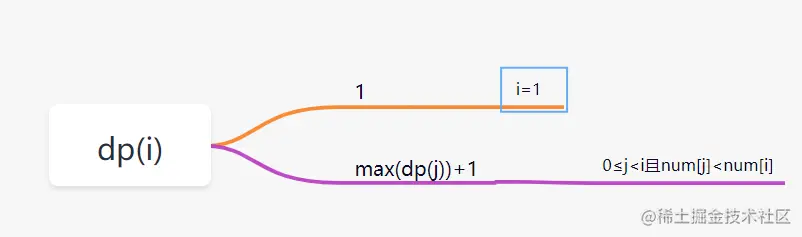``````最长递增子序列 =max(dp[i])
``````

#### 代码实现

``````class Solution {
public int lengthOfLIS(int[] nums) {
if (nums.length == 0) {
return 0;
}
int[] dp = new int[nums.length];
//初始化就是边界情况
dp = 1;
int maxans = 1;
//自底向上遍历
for (int i = 1; i < nums.length; i++) {
dp[i] = 1;
//从下标0到i遍历
for (int j = 0; j < i; j++) {
//找到前面比nums[i]小的数nums[j],即有dp[i]= dp[j]+1
if (nums[j] < nums[i]) {
//因为会有多个小于nums[i]的数，也就是会存在多种组合了嘛，我们就取最大放到dp[i]
dp[i] = Math.max(dp[i], dp[j] + 1);
}
}
//求出dp[i]后，dp最大那个就是nums的最长递增子序列啦
maxans = Math.max(maxans, dp[i]);
}
return maxans;
}
}
``````

• leetcode官网